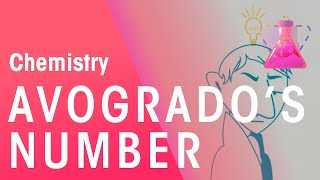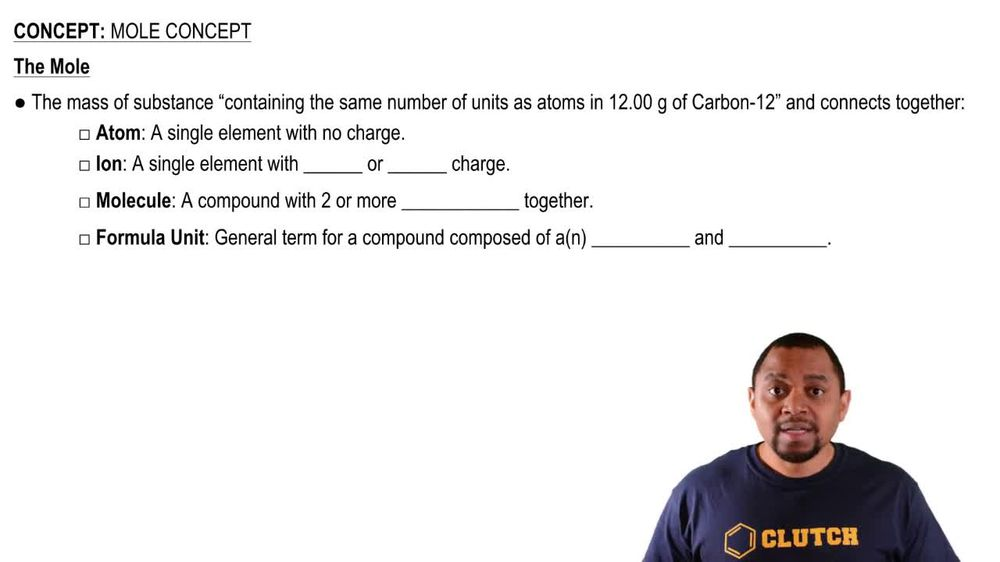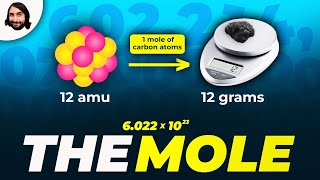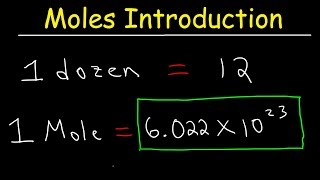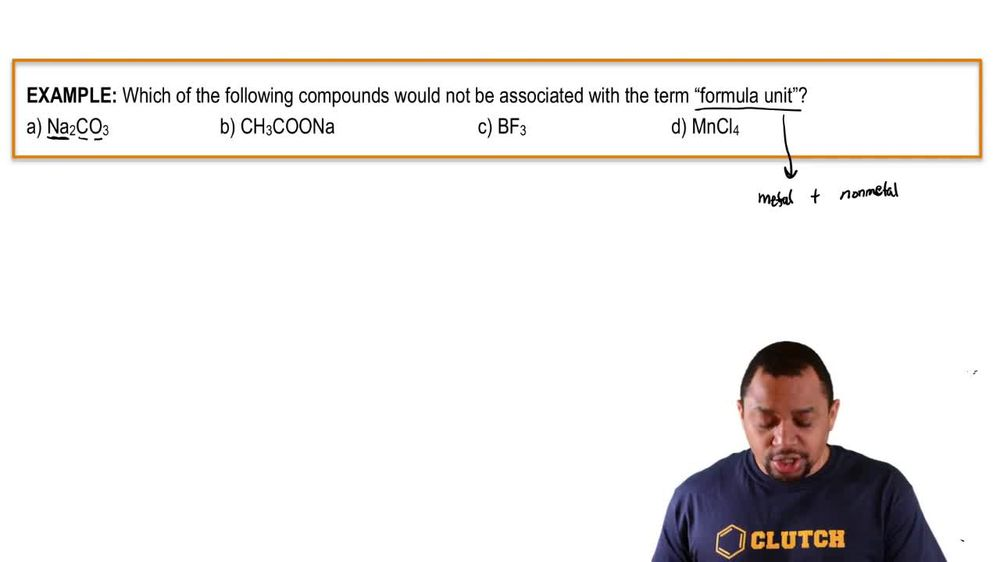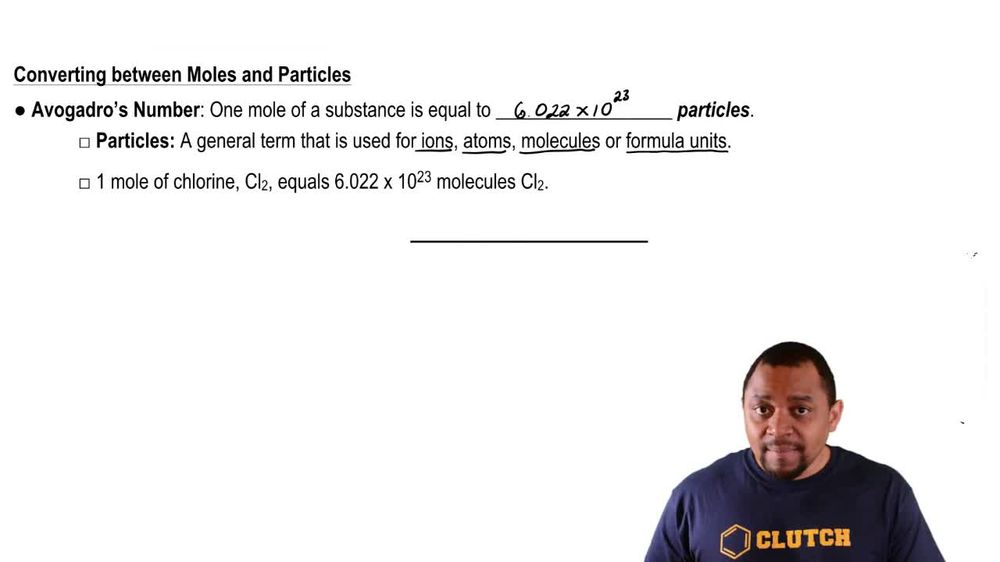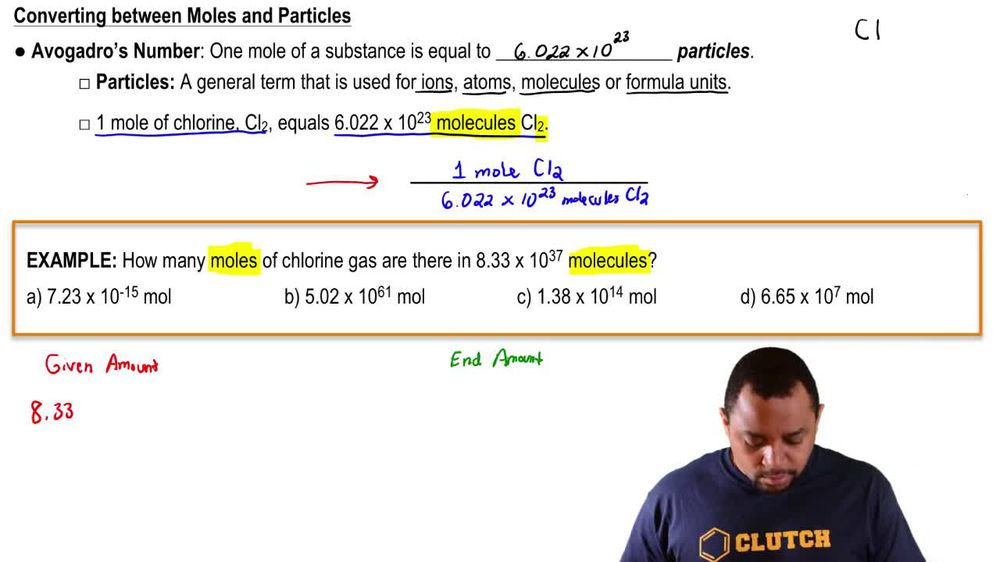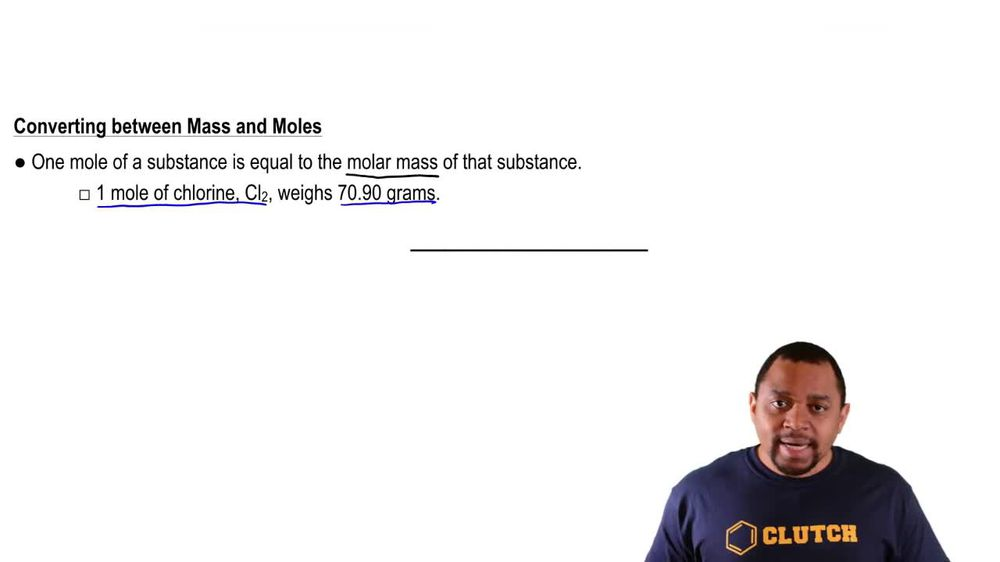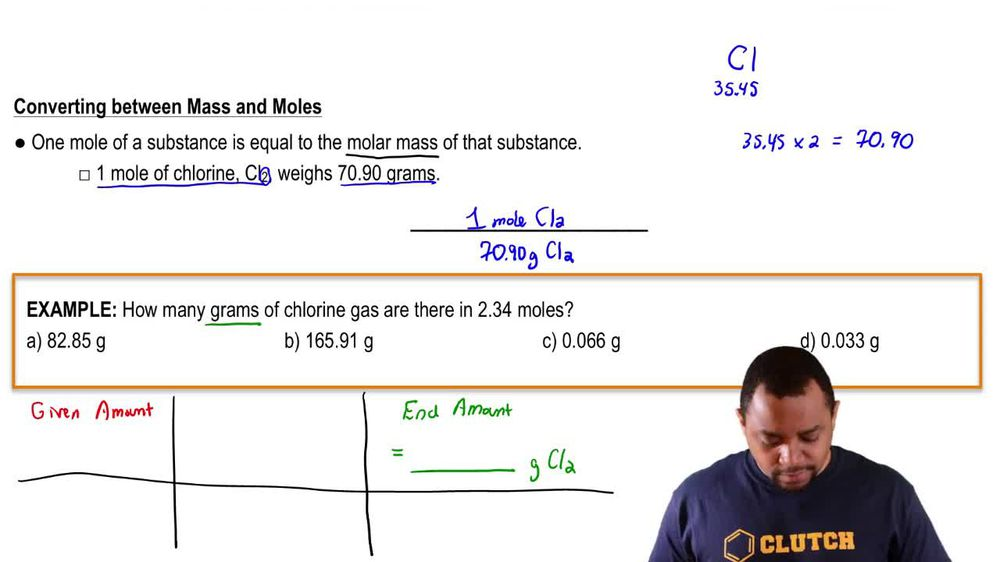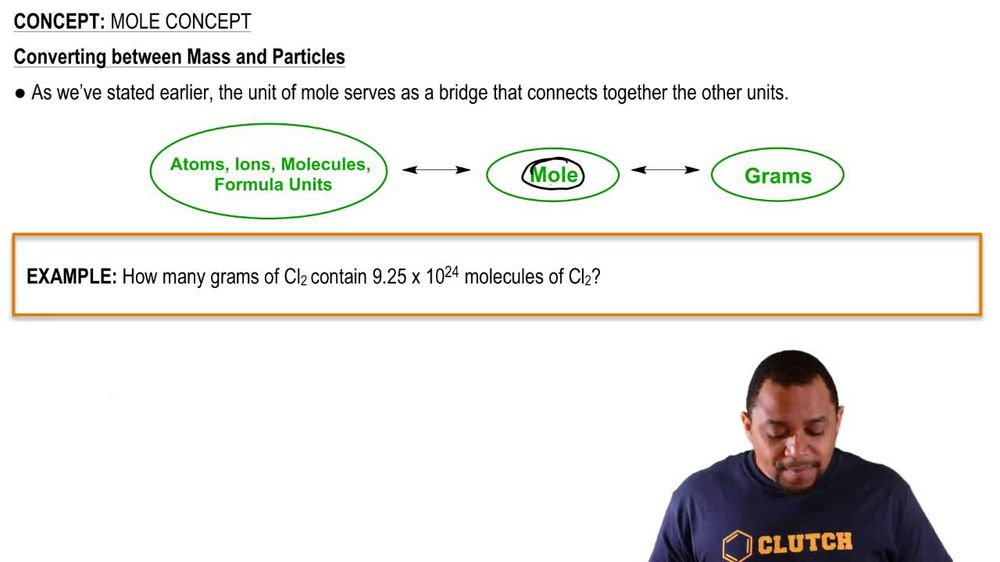Start typing, then use the up and down arrows to select an option from the list.
1. 2. Atoms & Elements2. Mole Concept
Problem

# Without doing any detailed calculations (but using a periodic table to give atomic weights), rank the following samples in order of increasing numbers of atoms: 0.5 mol BCl3 molecules, 197 g gold, 6.0 3 1023 CCl4 molecules.

Relevant Solution2m
Play a video:
Hey everyone were asked to rank the following samples in order of increasing numbers of atoms without doing calculations. So for our first one we have 3.5 mol boron tri chloride molecules. So here we have 3.5 moles But we also have four atoms per molecule. So without doing any calculations, this would mean that we have around 14 moles of atoms per molecule. Now let's go ahead and look at our second option, we have 269 g of titanium. Now looking at titanium molar mass, we know that this is 47.87 g per mole. So when we divide 269 g by 47.87, this gives us roughly around 5- moles. And since we only have one atom per mole, this gives us about 5-6 mole atoms in total. Looking at our last option, we have 7.84 times 10 to the 19th. Silicone tetra bromide molecules. Now looking at this value, this is much less than avocados number which is 6.22 times 10 to the 23rd and our exponents here is only 19, Which means that we have less than one mole of molecules. And since we have five atoms, we roughly have less than five moles of atoms. So when we rank these are least amount of Adams would be number three, which is our silicone tetra bromine molecules, followed by our titanium, which is our second option And our greatest one will be our boron tri chloride molecules and this will be our final answer. Now, I hope that made sense and let us know if you have any questions.